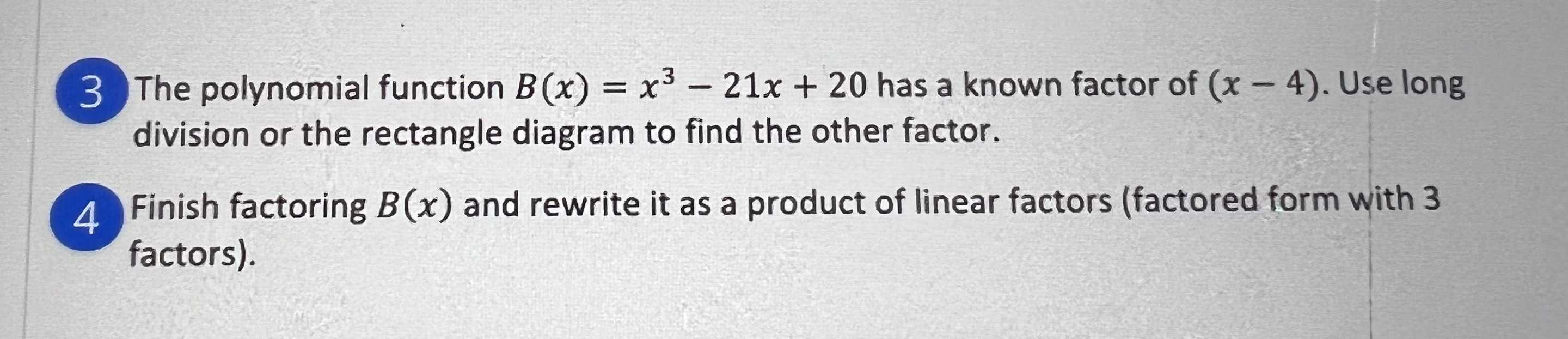### ¿Todavía tienes preguntas de matemáticas?

Pregunte a nuestros tutores expertos
Algebra
Pregunta(3. The polynomial function $$B ( x ) = x ^ { 3 } - 21 x + 20$$ has a known factor of $$( x - 4 )$$ . Use long division or the rectangle diagram to find the other factor.

(4.Finish factoring $$B ( x )$$ and rewrite it as a product of linear factors (factored form with $$3$$ factors).

3.   x$$^{2}$$+4x-5